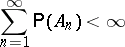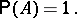# Difference between revisions of "Borel-Cantelli lemma"

2010 Mathematics Subject Classification: Primary: 60-01 Secondary: 60F1560F20 [MSN][ZBL]

A frequently used statement on infinite sequences of random events. Let \$A_1,\dots, A_n, \dots\$ be a sequence of events from a certain probability space and let \$A\$ be the event consisting in the occurance of (only) a finite number out of the events \$A_n\$, \$n=1,2\dots\$. Then, according to the Borel–Cantelli lemma, if(*)

thenIf the events \$A_n\$ are mutually independent, then \$\mathbb{P}(A) = 1\$ or \$0\$, depending on whether the seriesconverges or diverges, i.e. in this case the condition (*) is necessary and sufficient for; this is the so-called Borel criterion for "zero or one" (cf. Zero-one law). This last criterion can be generalized to include certain classes of dependent events. The Borel–Cantelli lemma is used, for example, to prove the strong law of large numbers.

How to Cite This Entry:
Borel-Cantelli lemma. Encyclopedia of Mathematics. URL: http://encyclopediaofmath.org/index.php?title=Borel-Cantelli_lemma&oldid=33512
This article was adapted from an original article by A.V. Prokhorov (originator), which appeared in Encyclopedia of Mathematics - ISBN 1402006098. See original article